# Normal distribution

(diff) ← Older revision | Latest revision (diff) | Newer revision → (diff)
Jump to: navigation, search

2010 Mathematics Subject Classification: Primary: 60E99 [MSN][ZBL]

One of the most important probability distributions. The term "normal distribution" is due to K. Pearson (earlier names are Gauss law and Gauss–Laplace distribution). It is used both in relation to probability distributions of random variables (cf. Random variable) and in relation to the joint probability distribution (cf. Joint distribution) of several random variables (that is, to distributions of finite-dimensional random vectors), as well as of random elements and stochastic processes (cf. Random element; Stochastic process). The general definition of a normal distribution reduces to the one-dimensional case.

The probability distribution of a random variableis called normal if it has probability density(*)

The family of normal distributions (*) depends, as a rule, on the two parametersand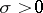. Hereis the mathematical expectation of,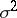is the variance ofand the characteristic function has the form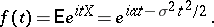The normal density curveis symmetric about the ordinate passing throughand has there its unique maximum. Asdecreases, the normal distribution curve becomes more and more pointed. A change inwith constantdoes not change the shape of the curve and causes only a shift along the-axis. The area under a normal density curve is 1. When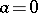and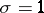, the corresponding distribution function is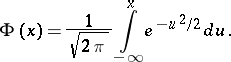In general, the distribution function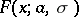of (*) can be computed by the formula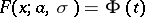, where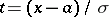. For(and several of its derivatives) extensive tables have been compiled (see, for example, [BS], [T], and Probability integral). For a normal distribution the probability thatisand it decreases very rapidly with increasing(see the Table).
<tbody> </tbody>
 k probability 1 0.31731 2 0.455003 0.269984 0.63342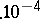In many practical problems, when analyzing normal distributions one can, therefore, ignore the possibility of a deviation fromin excess of— the three-sigma rule; the corresponding probability, as is clear from the Table, is less than 0.003. The quartile deviation for a normal distribution is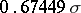.

Normal distributions occur in a large number of applications. There are some noteable attempts at explaining this fact. A theoretical basis for the exceptional role of the normal distribution is given by the limit theorems of probability theory (see also Laplace theorem; Lyapunov theorem). Qualitatively, the result can be stated in the following manner: A normal distribution is a good approximation whenever the relevant random variable is the sum of a large number of independent random variables the largest of which is small in comparison with the whole sum (see Central limit theorem).

A normal distribution can also appear as an exact solution of certain problems (within the framework of an accepted mathematical model of the phenomenon). This is so in the theory of random processes (in one of the basic models of Brownian motion). Classic examples of a normal distribution arising as an exact one are due to C.F. Gauss (the law of distribution of errors of observation) and J. Maxwell (the law of distribution of velocities of molecules) (see also Independence; Characterization theorems).

The distribution of a random vector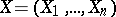in, or the joint distribution of random variables, is called normal (multivariate normal) if for any fixed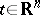the scalar producteither has a normal distribution or is constant (as one sometimes says, has a normal distribution with variance zero). For random elements with values from some vector spacethis definition is retained whenis replaced by any elementof the adjoint space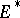and the scalar product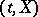is replaced by a linear functional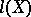. The joint distribution of several random variableshas characteristic function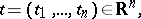where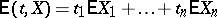is a linear form,is a non-negative definite quadratic form, and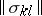is the covariance matrix of. In the positive-definite case the corresponding normal distribution has the probability density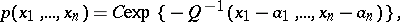where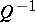is the quadratic form inverse to, the parametersare the mathematical expectations of, respectively, and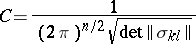is constant. The total number of parameters specifying the normal distribution isand grows rapidly with(it is 2 for, 20 for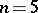, and 65 for). A multivariate normal distribution is the basic model of multi-dimensional statistical analysis. It is also used in the theory of stochastic processes (where normal distributions in infinite-dimensional spaces are examined; see Random element, and also Wiener measure; Wiener process; Gaussian process).

Of the important properties of normal distributions the following should be mentioned. The sumof two independent random variablesand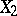having normal distributions also has a normal distribution; conversely, if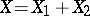has a normal distribution and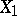andare independent, then the distributions ofandare normal (Cramér's theorem). This property has a certain "stability" : If the distribution ofis "close" to normal, then so are the distributions ofand. Some other important distributions are connected with normal ones (see Logarithmic normal distribution; Non-central "chi-squared" distribution; Student distribution; Wishart distribution; Fisher-distribution; Hotelling-distribution; Chi-squared distribution). For an approximate representation of distributions close to normal, series like Edgeworth series and Gram–Charlier series are widely used.

Concerning problems connected with estimators of parameters of normal distributions using results of observations see Unbiased estimator. Concerning testing the hypothesis of normality see Non-parametric methods in statistics. See also Probability graph paper.